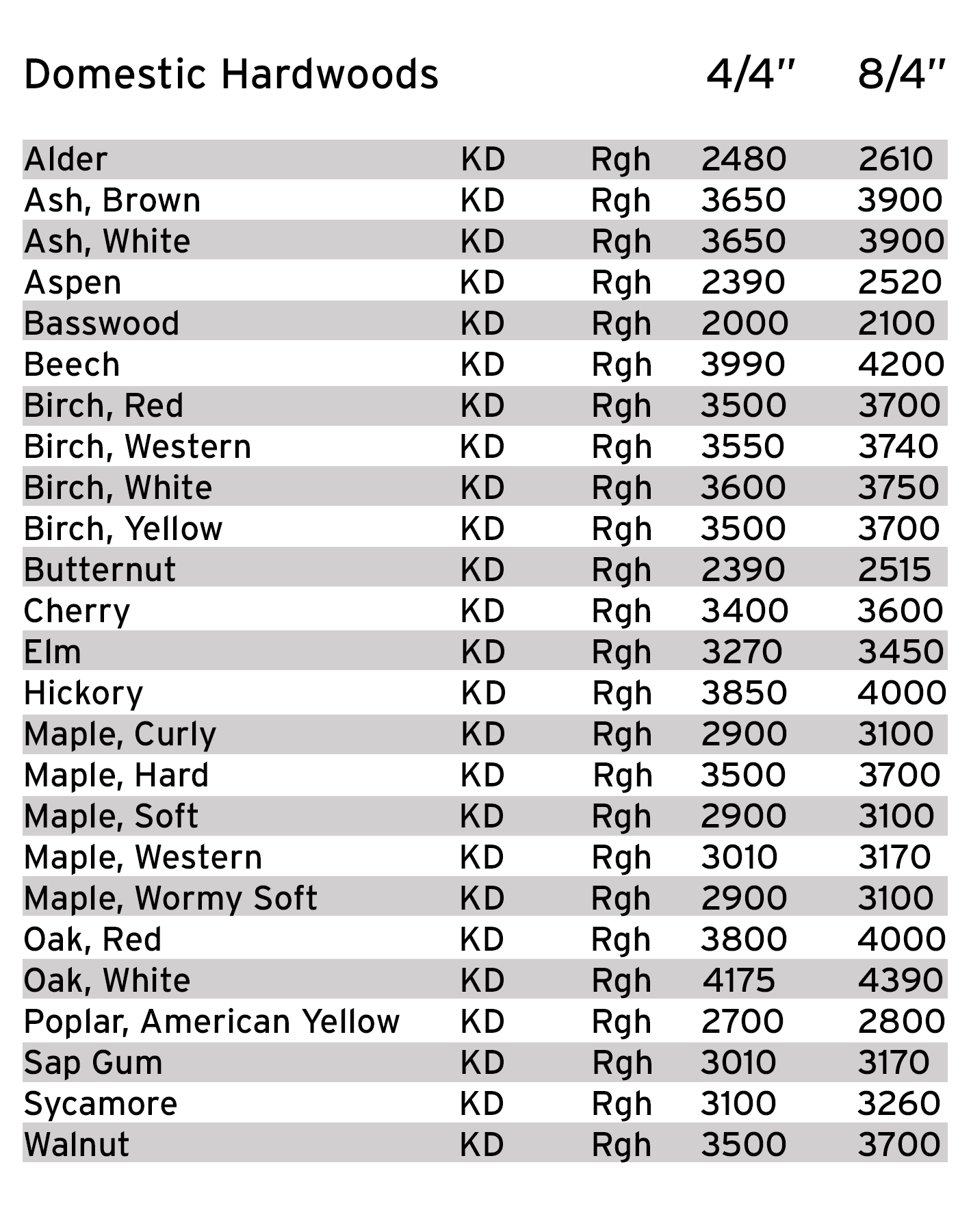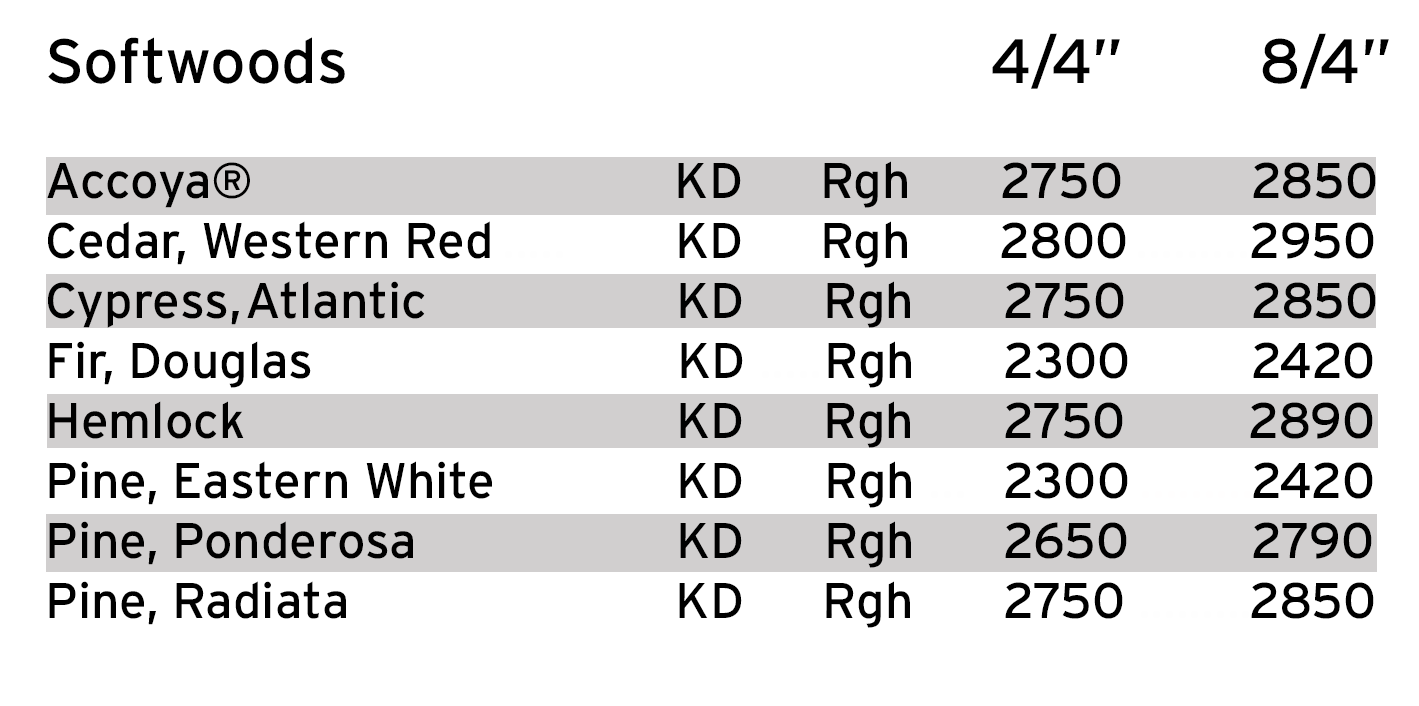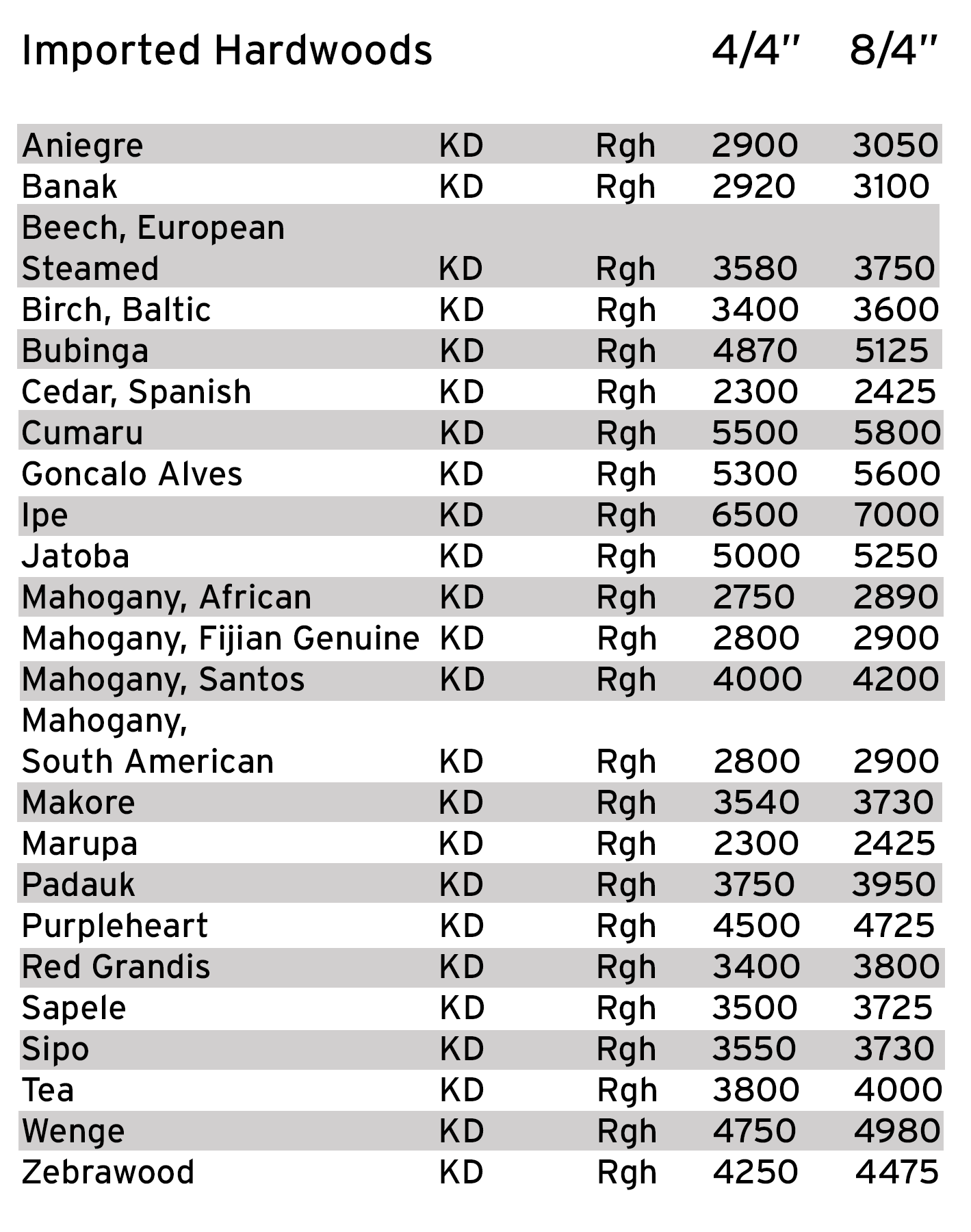# Conversion Formulas

Calculate lumber weights and footages

All calculations based on net footages

Calculate Board Feet (FBM) from Lineal Feet (LF)

FBM = LF x Width ÷ 12 x Thickness

Example
To convert 3,000 LF of 8" width x 1.25" thickness
Calculate 3,000 x 8 ÷ 12 x 1.25 = 2,500 FBM

PRICE/M FBM INTO PRICE/LF

Price/M ÷ 12 x Width x Thickness ÷ 1000 = Price/LF

Calculate Lineal Feet (LF) from Board Feet (FBM)

LF = FBM ÷ Width x 12 ÷ Thickness

Example
To convert 1,500 FBM of 6" width x 1.5" thickness
Calculate 1,500 ÷ 6 x 12 ÷ 1.5 = 2,000 LF

PRICE/LF into PRICE/M FBM

Price/LF x 12 ÷ Width ÷ Thickness x 1,000 = Price/M

Lumber Weights

Approx. Weight in lbs. per 1000 Board Feet